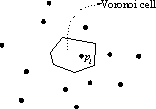## 1. Introduction

Voronoi diagram belong to classical problems of the computational geometry. Its origin dates back into 1850 when it was considered by Dirichlet. The rigid mathematical fundamental was given by Voronoi in 1908.

Let us have points pi, 0 < i <= n in n-dimensional space P. The set of all points with property that every point inside the cell is closer to point pi than to any other point from P represents the Voronoi cell (Figure 1). The union of all Voronoi cells is known as Voronoi diagram.Figure 1: Voronoi cell

As seen from Figure 1, the construction of Voronoi diagram consists of repetitive subdivisions of the space determined by the input points into subspaces to meet the Voronoi criteria:

In many aplications, Voronoi diagrams are already the final solution. For example, study of behavior and maintainance of live creature, which are depended on number of neighbors with whom they are fighting for food and lightness is exactly what Voronoi diagram expresses. However, having a Voronoi diagram, many important geometric features like searching for the closest neighbor, Delaunay triangulation, searching for the largest empty circle, minimum spanning tree (Euclidean tree), can be determined in the linear time.

The first reiable algorithm for construction has been published by Green and Sibson [GREE77], although the Voronoi diagrams have been known for a long time. This approach used incremental method and worked in O(n2) time. The first algorithm requiring O(n log2 n) was suggested by Shamos and Hoey (1975) [PREP85] using divide and conquer approach. However, its implementation is complicated. In 1985, Fortune presented more elegant approach and it is described in [BERG97, O'ROU93]. In this paper, we consider primarily the data structures needed for the construction. In 1986, Edelsbruner and Seidel discovered beautiful connection between Voronoi diagram and convex hulls in one higher dimension [O'ROU93]. This method has some beautiful properties and it is becoming more and more popular.

Roman Cuk - roman.cuk@uni-mb.si